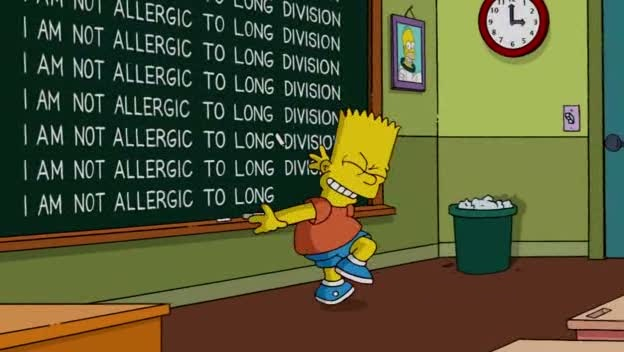# I am not allergic to long divisionDr. Richard P. Feynman posed the following puzzle: Each of the dots below represents some digit (any digit 0 to 9). Each of the $A$'s represents the same digit. None of the dots are the same as $A.$What is the sum of the divisor, the dividend, and the quotient?


Details and Assumptions:

• Both manual and CS solutions are encouraged.
• You can see the original letter below.×